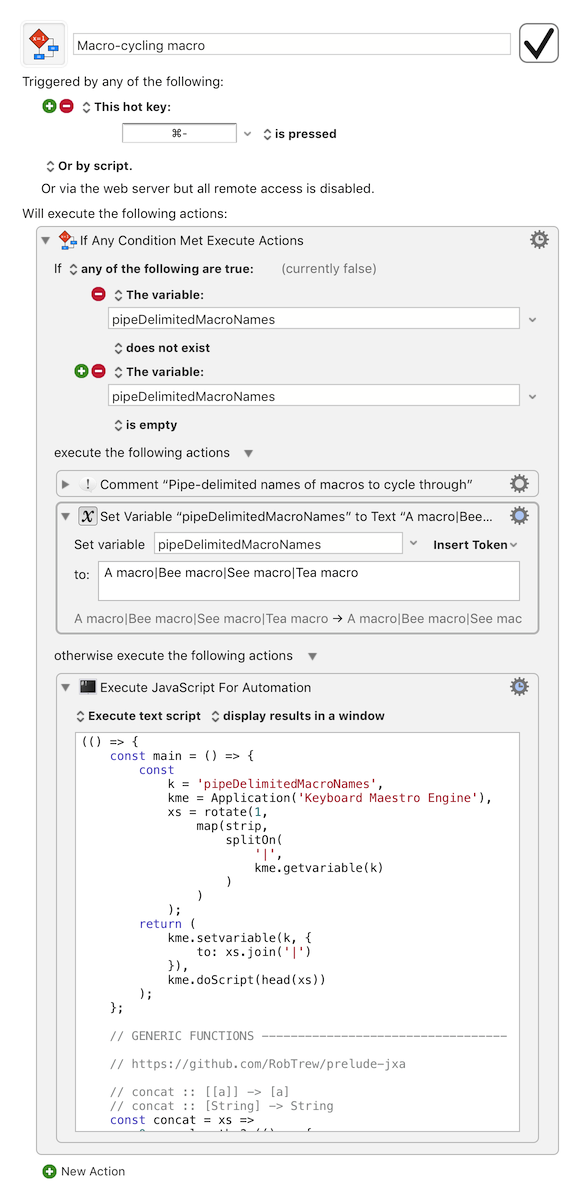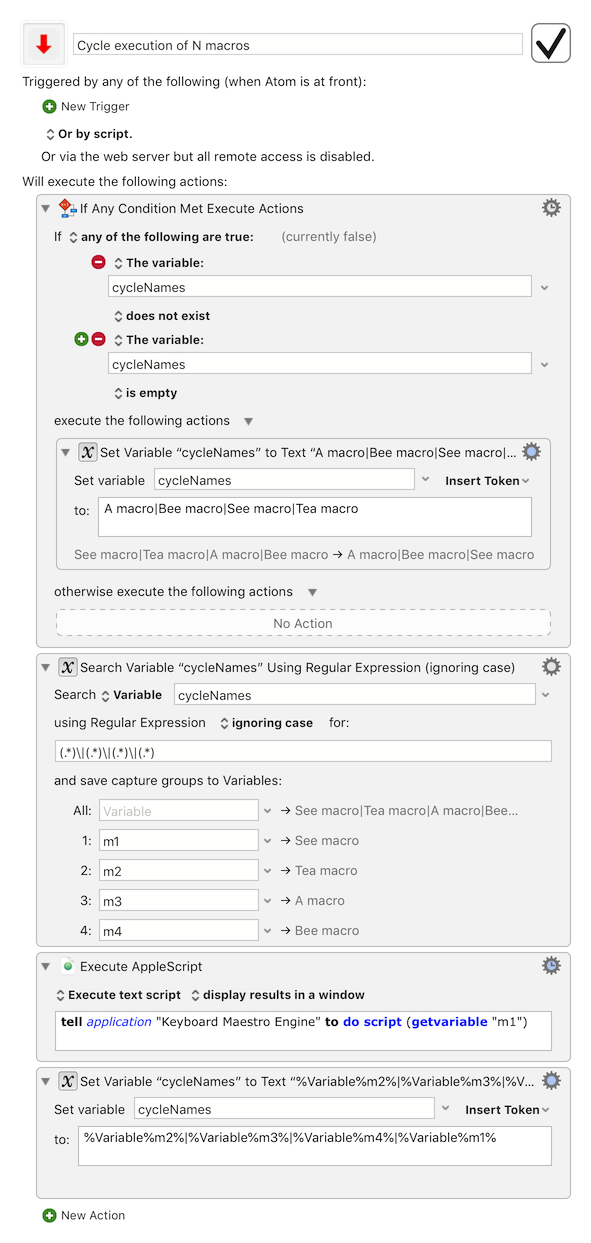# One hot-key to cycle through an arbitrary number of macros

Following up from an earlier extension from a two-macro cycle to a question about a three-macro cycle

Here is an approach to using one trigger to cycle through any number of macros.

Macro cycle Macros.kmmacros (10.0 KB)

Essentially you create a pipe-delimited text variable containing the name of each macro in the cycle, in the order that you need.

To cycle through 4 macros named:

• A macro
• Bee macro
• See macro, and
• Tea macro

you can create a variable called `pipeDelimitedMacroNames` in the following pattern:

`A macro | Bee macro | See macro | Tea macro`

(with or without spaces flanking the pipe characters)

and then run this meta-macro, in which a script step rotates the variable, and runs whichever macro's name has come to the front:Script source:

``````(() => {
const main = () => {
const
k = 'pipeDelimitedMacroNames',
kme = Application('Keyboard Maestro Engine'),
xs = rotate(1,
map(strip,
splitOn(
'|',
kme.getvariable(k)
)
)
);
return (
kme.setvariable(k, {
to: xs.join('|')
}),
);
};

// GENERIC FUNCTIONS ----------------------------------

// https://github.com/RobTrew/prelude-jxa

// concat :: [[a]] -> [a]
// concat :: [String] -> String
const concat = xs =>
0 < xs.length ? (() => {
const unit = 'string' !== typeof xs ? (
[]
) : '';
return unit.concat.apply(unit, xs);
})() : [];

// drop :: Int -> [a] -> [a]
// drop :: Int -> String -> String
const drop = (n, xs) => xs.slice(n);

// head :: [a] -> a
const head = xs => xs.length ? xs : undefined;

// map :: (a -> b) -> [a] -> [b]
const map = (f, xs) => xs.map(f);

// replicate :: Int -> a -> [a]
const replicate = (n, x) =>
Array.from({
length: n
}, () => x);

// rotate :: Int -> [a] -> [a]
const rotate = (n, xs) => {
const lng = xs.length;
return lng > 0 ? takeDropCycle(lng, n, xs) : [];
};

// splitOn :: String -> String -> [String]
const splitOn = (pat, src) =>
src.split(pat);

// strip :: String -> String
const strip = s => s.trim();

// take :: Int -> [a] -> [a]
const take = (n, xs) => xs.slice(0, n);

// N items taken from an infinite cycle of xs, starting from index i

// takeDropCycle :: Int -> [a] -> [a]
const takeDropCycle = (n, i, xs) => {
const
lng = xs.length,
m = n + i;
return drop(i,
take(m,
(lng >= m ? xs : concat(replicate(Math.ceil(m / lng), xs)))
)
);
};

// MAIN ---
return main();
})();

``````
1 Like

And an AppleScript variant of the macro list rotation script:

``````on run
set k to "pipeDelimitedMacroNames"

tell application "Keyboard Maestro Engine" to set strNames to getvariable k

set xs to rotate(1, map(strip, splitOn("|", strNames)))

tell application "Keyboard Maestro Engine"
setvariable k to my intercalateS("|", xs)
do script nextMacro
end tell
end run

-- GENERIC FUNCTIONS ------------------------------------------------

-- https://github.com/RobTrew/prelude-applescript

-- ceiling :: Num -> Int
on ceiling(x)
set nr to properFraction(x)
set n to |1| of nr
if (|2| of nr) > 0 then
n + 1
else
n
end if
end ceiling

-- concat :: [[a]] -> [a]
-- concat :: [String] -> String
on concat(xs)
set lng to length of xs
if 0 < lng and string is class of (item 1 of xs) then
set acc to ""
else
set acc to {}
end if
repeat with i from 1 to lng
set acc to acc & item i of xs
end repeat
acc
end concat

-- drop :: Int -> [a] -> [a]
-- drop :: Int -> String -> String
on drop(n, xs)
if class of xs is not string then
if n < length of xs then
items (1 + n) thru -1 of xs
else
{}
end if
else
if n < length of xs then
text (1 + n) thru -1 of xs
else
""
end if
end if
end drop

-- dropWhile :: (a -> Bool) -> [a] -> [a]
-- dropWhile :: (Char -> Bool) -> String -> String
on dropWhile(p, xs)
set lng to length of xs
set i to 1
tell mReturn(p)
repeat while i ≤ lng and |λ|(item i of xs)
set i to i + 1
end repeat
end tell
drop(i - 1, xs)
end dropWhile

-- dropWhileEnd :: (a -> Bool) -> [a] -> [a]
-- dropWhileEnd :: (Char -> Bool) -> String -> String
on dropWhileEnd(p, xs)
set i to length of xs
tell mReturn(p)
repeat while i > 0 and |λ|(item i of xs)
set i to i - 1
end repeat
end tell
take(i, xs)
end dropWhileEnd

-- head :: [a] -> a
if xs = {} then
missing value
else
item 1 of xs
end if

-- intercalateS :: String -> [String] -> String
on intercalateS(sep, xs)
set {dlm, my text item delimiters} to {my text item delimiters, sep}
set s to xs as text
set my text item delimiters to dlm
return s
end intercalateS

-- map :: (a -> b) -> [a] -> [b]
on map(f, xs)
tell mReturn(f)
set lng to length of xs
set lst to {}
repeat with i from 1 to lng
set end of lst to |λ|(item i of xs, i, xs)
end repeat
return lst
end tell
end map

-- min :: Ord a => a -> a -> a
on min(x, y)
if y < x then
y
else
x
end if
end min

-- Lift 2nd class handler function into 1st class script wrapper
-- mReturn :: First-class m => (a -> b) -> m (a -> b)
on mReturn(f)
if class of f is script then
f
else
script
property |λ| : f
end script
end if
end mReturn

-- properFraction :: Real -> (Int, Real)
on properFraction(n)
set i to (n div 1)
Tuple(i, n - i)
end properFraction

-- Egyptian multiplication - progressively doubling a list, appending
-- stages of doubling to an accumulator where needed for binary
-- assembly of a target length
-- replicate :: Int -> a -> [a]
on replicate(n, a)
set out to {}
if n < 1 then return out
set dbl to {a}

repeat while (n > 1)
if (n mod 2) > 0 then set out to out & dbl
set n to (n div 2)
set dbl to (dbl & dbl)
end repeat
return out & dbl
end replicate

-- rotate :: Int -> [a] -> [a]
on rotate(n, xs)
set lng to length of xs
if lng > 0 then
takeDropCycle(lng, n, xs)
else
{}
end if
end rotate

-- splitOn :: String -> String -> [String]
on splitOn(pat, src)
set {dlm, my text item delimiters} to ¬
{my text item delimiters, pat}
set xs to text items of src
set my text item delimiters to dlm
return xs
end splitOn

-- strip :: String -> String
on strip(s)
script isSpace
on |λ|(c)
set i to id of c
32 = i or (9 ≤ i and 13 ≥ i)
end |λ|
end script
dropWhile(isSpace, dropWhileEnd(isSpace, s))
end strip

-- take :: Int -> [a] -> [a]
-- take :: Int -> String -> String
on take(n, xs)
if class of xs is string then
if 0 < n then
text 1 thru min(n, length of xs) of xs
else
""
end if
else
if 0 < n then
items 1 thru min(n, length of xs) of xs
else
{}
end if
end if
end take

-- take N Members of an infinite cycle of xs, starting from index I
-- takeDropCycle :: Int -> [a] -> [a]
on takeDropCycle(n, i, xs)
set lng to length of xs
set m to n + i

if lng ≥ m then
set ys to xs
else
set ys to concat(replicate(ceiling(m / lng), xs))
end if

drop(i, take(m, ys))
end takeDropCycle

-- Tuple (,) :: a -> b -> (a, b)
on Tuple(a, b)
{type:"Tuple", |1|:a, |2|:b, length:2}
end Tuple
``````
1 Like

Thanks. It looks like the three macro trigger can be achieved with just Keyboard Maestro actions. But thanks for reply and method.

This is a really helpful forum!

Mark

1 Like

You asked a good question, which others may well search for again in time.

Worth leaving a range of solutions for them to find, I think : -)

and FWIW a marginally more Maestronic (tho also more regex-encumbered) version of the same approach:1 Like

Hey, Thank you for sharing that extremely useful macro! I'm using it with MIDI Knobs and I have a question about it. How should script look if I want to cycle back?In the script variants, (JS or AS) the first argument of the rotate function can be negative – to reverse the direction of the rotation. So while:

``````rotate(1, ["a", "b", "c", "d"])
``````

returns a right-cycled list

``````[
"d",
"a",
"b",
"c"
]
``````

in contrast:

``````rotate(-1, ["a", "b", "c", "d"])
``````

is left-cycled:

``````[
"b",
"c",
"d",
"a"
]
``````

Does that give you enough ?

1 Like

And for a non-script version, cycling a compound string of variable names to right or to left:

( Set the cycleBackward variable to a non-empty variable for a reversed cycle )

Cycle execution of N macros (LEFT or RIGHT).kmmacros (21.6 KB)1 Like

Thanks a lot!. I had an error with `rotate(-1, ...`, so I made macro with a line count filter. Macro Cycle - Left.kmmacros (9.1 KB)